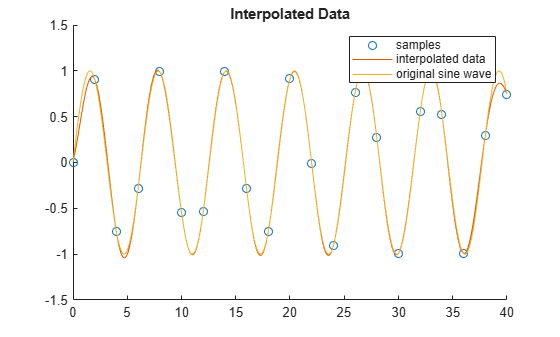# lowpassResample

Convert signal from one sample time to another

## Syntax

``[vq,vdq] = lowpassResample(t,v,tq,config)``

## Description

example

````[vq,vdq] = lowpassResample(t,v,tq,config)` returns an interpolated vector of samples `vq` and their derivatives `vdq` from input sample times `t`, input sample values `v`, and output sample times `tq`. The interpolation process is defined by the parameters in `config`.```

## Examples

collapse all

Sample a sine wave at pi samples per cycle.

```t = (0:20)*2; v = sin(t);```

Define the interpolation sample times.

`tq = (0:400)*0.1;`

Define the interpolation configuration.

```config.OutputRiseFall = 2; %Fixed step sample interval config.NDelay = 5; config.SampleMode = 'fixed'; config.CausalMode = 'off';```

Perform the interpolation.

`[vq,vdq]=lowpassResample(t,v,tq,config);`

Scatter plot the samples, plot the interpolated data, and plot the original sine wave.

```scatter(t,v); hold on; plot(tq,vq); plot(tq,sin(tq)); hold off; title('Interpolated Data'); legend('samples','interpolated data','original sine wave');```Plot the interpolated derivative and the original derivative.

```plot(tq,vdq); hold on; plot(tq,cos(tq)); hold off; title('Interpolated Derivative'); legend('interpolated derivative','original derivative');```## Input Arguments

collapse all

Input sample times, specified as a fixed-step or variable-step vector.

Data Types: `single` | `double` | `int8` | `int16` | `int32` | `uint8` | `uint16` | `uint32` | `logical`

Input sample values corresponding to the input sample times defined in `t`, specified as a vector.

Data Types: `single` | `double`

Output sample times, specified as a fixed-step or variable-step vector.

Data Types: `single` | `double`

Interpolation parameters, specified as a structure with fields.

FieldDescriptionValueDefault
`OutputRiseFall`The 0%–100% rise/fall time of the interpolated output.Positive real scalar`1e-10`
`NDelay`Number of rise/fall times by which the interpolated output will be delayed with respect to the input.Positive real integer`1`
`SampleMode`Input sampling mode, either fixed-step discrete time or variable-step discrete time.`fixed`, `variable``variable`
`CausalMode`Determine whether you want the interpolation process to introduce delay. Select `CausalMode` to introduce enough delay between input and output samples in the interpolation process so that the process is strictly causal.`off`, `on``off`

Data Types: `struct`

## Output Arguments

collapse all

Interpolated samples, returned as a vector.

Data Types: `single` | `double`

Derivative of interpolated samples corresponding to `vq`, returned as a vector.

Data Types: `single` | `double`

collapse all

### Delay in Interpolated Output

You can define the number of rise/fall times by which the interpolated output will be delayed with respect to the input using the `config.NDelay` parameter.

The default value of `config.NDelay` of 1 produces an interpolation that has no ringing due to the Gibbs phenomenon and also has modest rejected out of band numerical artifacts.

Setting `config.NDelay` to 5 introduces enough anti-aliasing filtering to satisfy most applications, but introduces ringing due to the Gibbs phenomenon.

Setting `config.NDelay` to 10 introduces enough anti-aliasing filtering to satisfy demanding applications, but at the cost of additional delay and computation.

Setting `config.NDelay` to greater than 10 is supported, but is not required normally.

### Input Sampling Mode

You can define the input sampling mode using the `config.SampleMode`parameter.

The default value of `config.SampleMode` of `variable` assumes that the input values are the result of a zero order hold process. In this case, the signal value is always equal to the value of the most recent sample. This choice is appropriate for saturated signals for which the transition times are the most important consideration.

Setting '`config.SampleMode` to `fixed` assumes that the input values are instantaneous samples of a mathematically continuous signal at uniformly spaced sample times. Use this option for signals that are subject to a significant amount of analog filtering.

### Causality of Interpolation Process

You can define the interpolation process as causal or not using the `config.SampleMode` parameter.

The default value of `config.CausalMode` of `off` aligns the time scale of the interpolation with the time scale of the input. This is appropriate when all necessary input values are available in a single vector.

Setting `config.CausalMode` to `on` introduces enough delay so that the sample values are available before performing an interpolation. The interpolated sample times are therefore always delayed by a constant value with respect to the input sample times. The required additional delay in this mode is `config.OutputRiseFall` times `config.NDelay`. This behavior mimics the behavior of the Lowpass Resampler block.

## Version History

Introduced in R2021a